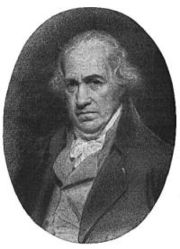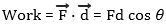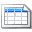# Back to basics - the Watt (or kW)

By on

 When thinking about watts (W) or kilowatt (kW = 1000 W) it can be useful too keep in mind the fundamental ideas behind the unit. Watt is not a pure electrical or mechanical unit, but is a measure of the rate of doing work. Let start at the beginning and understand the meaning of work.  This is effectively what the word says; doing work involves doing something - like moving things from one place to the other, climbing stairs, etc. In physics this is equated to the energy used in in transferring a force through a distance in the direction of the force. The SI unit of work is the Joule. Another more general way to look at work it to consider that if the force acting on an object changes it's kinetic energy then the work done is equal to the change in kinetic energy.  So does work = force x distance? Sometimes. Work is a scalar quantity and this equation holds if the force is acting in the same direction as the movement. If the force and distance are not acting in the same direction then we need to take the dot product of the force and distance vectors:James Watt 19 January 1736 - 19 August 1819If the angle θ is zero the work is simply the force multiplied by distance If the force is perpendicular to the distance, cos θ = 0 and no work is done.

With an understanding of work, we can get back to the main topic - Watts.  This is simply a measure of the rate at which work is carried out,  i.e.:

1 Watt = 1 Joule of work per Second

What is the rate of doing work? The answer is Power.  If we want to measure the power of a car or electrical motor we want to look at the amount of energy it can deliver every second (i.e. Watts).    The unit Watt is named after the Scottish engineer James Watt (famous for his work on steam engines).

With an understanding of the concepts,  an example will tie everything together:

Example:  a 50 kG weight is lifted vertically 20 m in 10 seconds (with the lifting force acting in the same direction as the movement):

Work done = 50  x 20 = 1,000 Joules

The work is done over 10 seconds, so the average power (rate of using energy) is:

Power = 1,000 / 10 = 100 Watts

That’s  it.  Before finishing a few final observations:

• 1 kW (kilowatt) is 1000 W (Watts).   Watt is the SI unit or power, although in the United States horse power (HP) as a unit is still common.  One HP is nominally the amount of energy a horse could deliver each second.  As each real horse is different this has been standardised to the Watt, with  1 HP  = 764 Watts
• kWHr (kilowatt-hours) is the power multiplied by the time it is used. For example if 1 kW is used for 2 hours than 2 kWHr is used  (or 2,000 W x 7,200 seconds = 14.4 10^6 Watt-Seconds). Form the above, 1 watt-second is 1 Joule (i..e the energy expended).  kWHr measures energy and not power

More interesting Notes:Steven McFadyen

Steven has over twenty five years experience working on some of the largest construction projects. He has a deep technical understanding of electrical engineering and is keen to share this knowledge. About the author

Load Flow Study – how they work

A load flow study is the analysis of an electrical network carried out by an electrical engineer. The purpose is to understand how power flows around...

Understanding Circuit Breaker Markings

IEC 60947 is the circuit breaker standard and covers the marking of breakers in detail. Any manufacturer following this standard should comply with the...

Why a Sine Wave?

I received this question by email a few weeks. First thoughts was that it is a product of the mathematics of rotating a straight conductor in a magnetic...

Cable Sheath and Armour Loss

When sizing cables, the heat generated  by losses within any sheath or armour need to be evaluated. When significant, it becomes a factor to be considered...

Capacitor Theory

Capacitors are widely used in electrical engineering for functions such as energy storage, power factor correction, voltage compensation and many others...

What is Aircraft Ground Power

Ever wondered what kind of power an aircraft uses when parked at the airport stand. Normally the aircraft generates it own power, but when parked with...

Nikola Tesla

Nikola Tesla was born exactly at midnight on July 10, 1856 in the tiny village of Smiljan, Lika in Croatia. In his late teens, Tesla left the village to...

Back to basics - the Watt (or kW)

When thinking about watts (W) or kilowatt (kW = 1000 W) it can be useful too keep in mind the fundamental ideas behind the unit. Watt is not a pure electrical...

Robotics - Home Innovations

We have a sister note to this (Robots - Interesting Video), in which I have posted some videos of interesting robots developed by commercial corporations...

8 Steps to Low Voltage Power Cable Selection and Sizing

A recurring theme on our forums is cable sizing. Now many installations are unique and require special consideration. However, a lot of the time things...## Have some knowledge to share

If you have some expert knowledge or experience, why not consider sharing this with our community.

By writing an electrical note, you will be educating our users and at the same time promoting your expertise within the engineering community.

To get started and understand our policy, you can read our How to Write an Electrical NoteClick here to view the notes list in a table formatClick here to view the notes indexed by tag